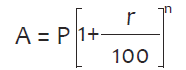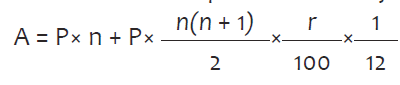Java Programs

Program 

Write a program to input an integer and check whether it is greater than 100, or less than 100 or equal to 100.

Program 

Write a program to input the Selling Price and Cost Price of a commodity and find the Profit or Loss made upon selling the product.

For example,

INPUT:

Enter the Selling Price of the commodity: 456

Enter the Cost Price of the commodity: 400

OUTPUT:

Profit: 56

INPUT:

Enter the Selling Price of the commodity: 300

Enter the Cost Price of the commodity: 310

OUTPUT:

Loss: 10

Program 

Write a program to check whether a int type number taken as input is a positive, negative or a zero.

Program 

Write a program to check whether a short type number taken as input is an even number or an odd number.

Program 

Write a program to accept an int type value as a parameter and print the square of it, if it is more than 120, otherwise print its cube.

Program 

Write a program to input 5 real numbers(floating point) and find their average. If the average is greater than 50 print good, otherwise print bad.

Program 

Write a program to check whether an int type number (taken as input) is a multiple of 5 or not.

Program 

Write a program to check whether an int type number (taken as input) is a 2-digit number or not.

Program 

Write a program to check whether an int type number (taken as input) is a 3-digit number divisible by three or not.

Program 

Write a program to input 2 integers and check whether both the numbers are multiples of 7 or not.

Program 

Write a program to input three integers and check whether all of them are unique (different) from each other or not.

Program 

Write a program to pass 2 integer numbers as parameters. If either of the two numbers is 0, display invalid entry and the program should end, if it is valid entry, divide the larger number with the smaller number and display the result.

Program 

Write a program to accept three sides of a triangle as parameter and check whether it can form a triangle or not. If it forms a triangle, check whether it is an acute angled, obtuse angled or right-angled triangle.

(Hint: To form a triangle, each side should be less the sum of the other two sides..

To form an acute angled triangle the square of every side should be less than the sum of the squares of the other two sides.

To form an obtuse angled triangle the square of any side should be greater than the sum of the squares of the other two sides.

To form an right angled triangle the square of any side should be equal to the sum of the squares of the other two sides.)

Program 

Write a program to accept a mark obtained by a student in computer science and print the grades accordingly:

 Marks Grade Above 90 A 70 to 89 B 50 to 69 C below 50 D

Program 

A cloth showroom has announced the following festival discounts on the purchase of items, based on the total cost of the items purchased:

 Total Cost Discount (in Percentage) Less than ₹ 2000 5% ₹ 2001 to ₹ 5000 25% ₹ 5001 to ₹ 10000 35% Above ₹ 10000 50%

Write a program to input the total cost and compute and display the amount to be paid by the customer after availing the discount.

Program 

An electricity company charges their consumers according to the units consumed per month according to the given traffic:

 Units Consumed Charges Up to 100 units ₹ 2 per unit More than 100 units and up to 200 units ₹ 1.80 per unit More than 200 units ₹ 1.50 per unit

In addition to the above, every consumer has to pay ₹ 200 as Service Charge per month. Write a program to input the amount of units consumed and calculate the total charges payable (Bill) by the consumer.

Program 

A library charges a fine for books returned late. Following are the fines:

First five days : 40 paise per day.

Six to Ten days : 65 paise per day.

Above ten days : 80 paise per day.

Design a program to calculate the fine assuming that a book is returned N days late.

Program 

Write a program to input an integer and check whether it is a 5-digit number or not. If it is extract the central digit and print it.

Example

INPUT: Enter an integer: 76549

OUTPUT: Central digit: 5

INPUT: Enter an integer: 12976

OUTPUT: Central digit: 9

INPUT: Enter an integer: 126

OUTPUT: Not a 5 digit number

Program 

Write a program to input 3 unique integers and print the smallest among them

Program 

Write a program to input the three angles of a triangle and check whether it forms a triangle or not, if it forms a triangle, check whether it is an equilateral, isosceles or a scalene triangle.

(Hint: To form a triangle, the sum of the angles should be 180 degrees.

To form an equilateral triangle every angle should be equal.

To form an isosceles triangle any two angles should be equal.

To form a scalene triangle all three angles should be different from each other.)

Program 

Write a program to input the three sides of a triangle and check whether it forms a triangle or not, if it forms a triangle, check whether it is an equilateral, isosceles or a scalene triangle.

(Hint: To form a triangle, each side should be less the sum of the other two sides.

To form an equilateral triangle every side should be equal.

To form an isosceles triangle any two sides should be equal.

To form a scalene triangle all three sides should be different from each other.)

Program 

Write a program to accept three sides of a triangle as parameter and check whether it can form a triangle or not. If it forms a triangle, check whether it is an acute angled, obtuse angled or right-angled triangle.

(Hint: To form a triangle, each side should be less the sum of the other two sides..

To form an acute angled triangle the square of every side should be less than the sum of the squares of the other two sides.

To form an obtuse angled triangle the square of any side should be greater than the sum of the squares of the other two sides.

To form an right angled triangle the square of any side should be equal to the sum of the squares of the other two sides.)

Program 

A special two-digit number is such that when the sum of its digits is added to the product of

its digits, the result is equal to the original two-digit number.

Example: Consider the number 59.

Sum of digits = 5 + 9 = 14

Product of its digits = 5 x 9 = 45

Sum of the sum of digits and product of digits = 14 + 45 = 59

Write a program to accept a two-digit number. Add the sum of its digits to the product of its

digits.

If the value is equal to the number input, output the message “Special 2-digitnumber” otherwise,

output the message “Not a special2-digit number”.

Program 

Using the switch statement, write a menu driven program to calculate the maturity amount of a Bank Deposit.

The user is given the following options:

i. Term Deposit

ii. Recurring Deposit

For option (i) accept principal(P), rate of interest(r) and time period in years(n). Calculate and output the maturity amount(A) receivable using the formulaFor option (ii) accept Monthly Instalment (P), rate of interest(r) and time period in months(n). Calculate and output the maturity amount(A) receivable using the formulaFor an incorrect option, an appropriate error message should be displayed.

Program 

Write a program in Java to accept three numbers and check whether they are  Pythagorean triples or not. The program must display the message accordingly .

A "Pythagorean Triple" is a set of positive integers, a, b and c that fits the rule:

a2 + b2 = c2

Program 

Write a Java program to input a number form user and check whether number is buzz number or not.

A number is said to be Buzz Number if it ends with 7 or is divisible by 7.